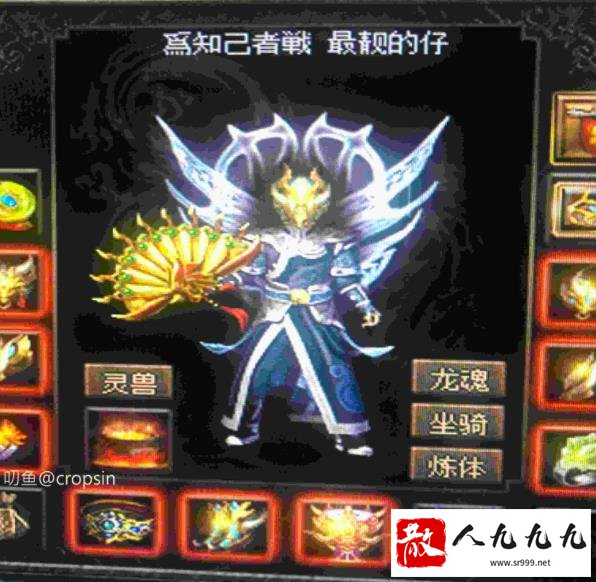# 武器熔炼升级脚本

 最近好多人咨询怎么升级武器，我都不知道怎么回答，我怕失败之后玩家找我，现在我把武器的升级脚本及公示公开给大家，以便大家研究。注意事项： 武器熔炼规则定义   localtForgeRule= { PayGold=100000,--需要支付10WG币 PayOre=--需要支付矿石 { Type=5,--矿石类型 SubType=0,--矿石子类型 Puritys=--不同矿石纯度贡献值 { 黑铁矿石1=1, 黑铁矿石2=2, 黑铁矿石3=3, 黑铁矿石4=4, 黑铁矿石5=5, 黑铁矿石6=6, 黑铁矿石7=7, 黑铁矿石8=8, 黑铁矿石9=9, 黑铁矿石10=10, 黑铁矿石11=11, 黑铁矿石12=12, 黑铁矿石13=13, 黑铁矿石14=14, 黑铁矿石15=15, 黑铁矿石16=16, 黑铁矿石17=17, 黑铁矿石18=18, 黑铁矿石19=19, 黑铁矿石20=20 }, DurabilityRate=15,--耐久基准阀值15,暂定公式:最终当前耐久=当前耐久*(当前纯度/(DurabilityRate*熔炼次数)); }, PayItem=--需要支付首饰 { Types={1,1,1},--支付物品{1,3}=手镯 SubTypes={3,8,9}, AttrRate=80,--首饰的属性总和阀值80,暂定公式:最终最大总和=(装备最大攻击*AttrRate)+熔炼次数; }, ForgeItem= { ForgeLevel=7,--最多熔炼7次 ForgeRate={--熔炼基准阀值(0-10000),暂定公式:最终成功概率=当前成功率概+(当前成功率概*(当前纯度/(DurabilityRate*熔炼次数))/10); 5000,--如:第一次强化放入3个15级黑矿,则成功概率=8000+(8000*(45/(15*1))/10)=10400=100% 4000,--如:第二次强化放入3个15级黑矿,则成功概率=6000+(6000*(45/(15*2))/10)=6900=69% 3000,--如:第三次强化放入3个15级黑矿,则成功概率=5000+(5000*(45/(15*3))/10)=5500=55% 2000, 1000, 500, 300,--如:第7次强化放入10个15级黑矿,则成功概率=1000+(1000*(150/(15*7))/10)=1142=%11.4 0--如:第8次永远为0 }, ForgeInc=2,--增量,一次最大攻击涨一点 ForgeCD=5--熔炼CD时间 } }